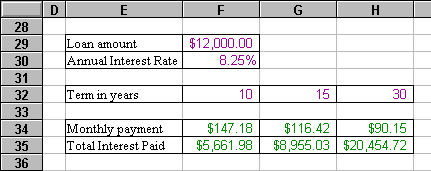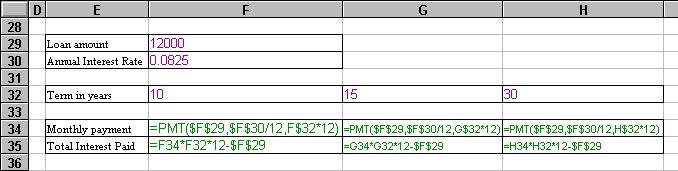## Concepts

### How are relative references different from absolute references?

You already know how to refer to a cell by its row-and-column position, such as A1 or E27. Written like that, these are called relative references.

When a formula is copied from one cell to another, any relative references contained in it are adjusted to bear the same relationship to the new formula location as they did to the old. For example, if cell C8 contains the formula =SUM(C1:C7) and you copy from cell C8 to D8, cell D8 will get the formula =SUM(D1:D7). If you copy the same formula to E67, it will become =SUM(E60:E66).

An absolute reference, on the other hand, is copied verbatim. The formula =SUM(\$C\$1:\$C\$7) in C8 remains =SUM(\$C\$1:\$C\$7) when copied to E67 (Or anywhere else).

The \$ in before the column letter indicates an absolute, or fixed, reference to that column, and the \$ before the row number is an absolute row reference.

References may also be mixed, such as \$C8 (fixed column, relative row) or C\$8 (relative column, fixed row).

Read on for an example of a spreadsheet that uses both reference types....

### When do you use absolute references?

You'll want to use an absolute reference whenever you're referring to one of those "reference values" we talked about earlier – values that are unique within the spreadsheet but may be used by formulas in many different places in the spreadsheet.

For example:Here is a simple spreadsheet showing the effect of varying loan terms on a \$12,000 loan with an interest rate of 8.25%. This spreadsheet contains three distinct kinds of information:

Loan Amount and Annual Interest Rate are single, reference values.

Term in years is a row of several alternate values. We're asking: "What happens if I pay back this loan over a ten-year term? A fifteen-year term? Thirty years?" Below each possible term we're building a related column of computed information.

Monthly Payment and Total Interest Paid are computed values, based on the reference values and on one of the possible loan terms.

Let's look at the formulas used in these computations.First, look at the formula in cell F34. We're using the PMT function to compute the monthly payment for the loan with a ten-year term.

Note that \$F\$29 is an absolute reference, because Loan Amount is always in cell F29. The same is true for Interest Rate in cell F30. Now notice that F\$32 refers to the row absolutely, but to the column relatively. This is because Term in Years is always in row 32, but its various values (10, 15, 30) use multiple columns.

Having written our payment computation this way, we can now Copy or Fill this formula into the cells to the right: G34 and H34. When copied, the absolute references continue to point to the unchanging values and the F\$32 becomes G\$32 and H\$32 to use the appropriate loan term value for each column.

The Total Interest Paid computation in F35 makes relative reference to cells F34 and F32. (F\$34 and F\$32 would be somewhat more proper, but since we'll only be copying the formula to the right – not right and down – it isn't crucial that the row references be absolute.) Just as we did with the Monthly Payment formula, we can Copy or Fill our Total Interest Paid formula to the right and each copy will adjust itself to refer to the correct data.

Why is it important to know how to use both relative and absolute references?

1. If you set up your formulas' cell references correctly, they will continue to function correctly even if you later add, delete, or move rows and columns in the process of building your spreadsheet.

2. If you set them up correctly, you can replicate formulas anywhere in the spreadsheet, using Copy/Paste or Fill, and they will behave as you intend them to.

Copyright 1997 by the Curators of the University of Missouri